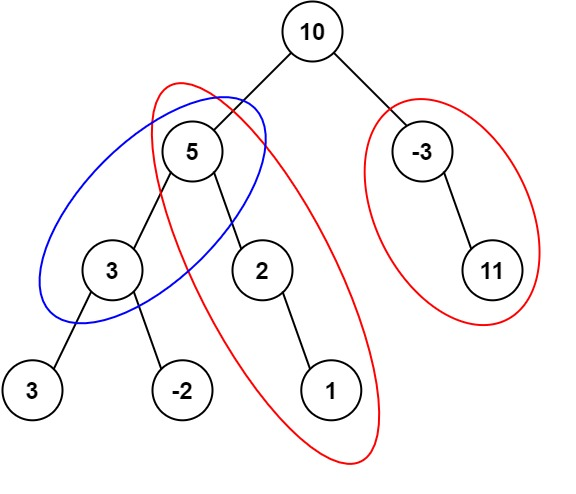# Leetcode 437 - Path Sum III

Note:

•• Path sum on a tree, usually we need DFS.
• What should we return in each DFS?
• It should include node.val, and add node.val to every combination from children.
• Base case: Return [].
• What to do in each recursion?
• If node.val === target, ans++;
• Put left and right results together, and see how many target - val are in there.
• Return [val, ...left.map(e => e + val), ...right.map(e => e + val)];
• Basically, preSum of children nodes.

Question:

Given the root of a binary tree and an integer targetSum, return the number of paths where the sum of the values along the path equals targetSum.

The path does not need to start or end at the root or a leaf, but it must go downwards (i.e., traveling only from parent nodes to child nodes).

Example:Code: